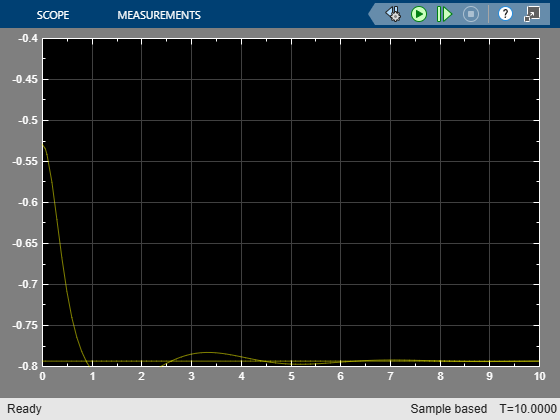# Design Model Predictive Controller at Equilibrium Operating Point

This example shows how to design a model predictive controller with nonzero nominal values.

The plant model is obtained by linearization of a nonlinear plant in Simulink® at a nonzero steady-state operating point.

### Linearize Nonlinear Plant Model

```if ~mpcchecktoolboxinstalled('simulink') disp('Simulink is required to run this example.') return end if ~mpcchecktoolboxinstalled('slcontrol') disp('Simulink Control Design is required to run this example.') return end ```

The nonlinear plant is implemented in Simulink model `mpc_nloffsets` and linearized at the default operating condition using the `linearize` function from Simulink Control Design.

Create operating point specification for the current model initial condition.

```plant_mdl = 'mpc_nloffsets'; op = operspec(plant_mdl); ```

Compute the operating point for this initial condition.

```[op_point, op_report] = findop(plant_mdl,op); ```
``` Operating point search report: --------------------------------- Operating point search report for the Model mpc_nloffsets. (Time-Varying Components Evaluated at time t=0) Operating point specifications were successfully met. States: ---------- (1.) mpc_nloffsets/Integrator x: 0.575 dx: -1.82e-14 (0) (2.) mpc_nloffsets/Integrator2 x: 2.15 dx: -8.38e-12 (0) Inputs: ---------- (1.) mpc_nloffsets/In1 u: -1.25 [-Inf Inf] Outputs: ---------- (1.) mpc_nloffsets/Out1 y: -0.529 [-Inf Inf] ```

Extract nominal state, output, and input values from the computed operating point.

```x0 = [op_report.States(1).x;op_report.States(2).x]; y0 = op_report.Outputs.y; u0 = op_report.Inputs.u; ```

Linearize the plant at the initial condition.

```plant = linearize(plant_mdl,op_point); ```

### Design MPC Controller

Create an MPC controller object with a specified sample time `Ts`, prediction horizon `p`, and control horizon `m`.

```Ts = 0.1; p = 20; m = 3; mpcobj = mpc(plant,Ts,p,m); ```
```-->The "Weights.ManipulatedVariables" property of "mpc" object is empty. Assuming default 0.00000. -->The "Weights.ManipulatedVariablesRate" property of "mpc" object is empty. Assuming default 0.10000. -->The "Weights.OutputVariables" property of "mpc" object is empty. Assuming default 1.00000. ```

Set the nominal values in the controller.

```mpcobj.Model.Nominal = struct('X',x0,'U',u0,'Y',y0); ```

Set the output measurement noise model (white noise, zero mean, variance = 0.01).

```mpcobj.Model.Noise = 0.1; ```

Set the manipulated variable constraint.

```mpcobj.MV.Max = 0.2; ```

Specify the reference value for the output signal.

```r0 = 1.5*y0; ```

Open and simulate the model.

```mdl = 'mpc_offsets'; open_system(mdl) sim(mdl) ```
```-->Converting model to discrete time. -->Assuming output disturbance added to measured output channel #1 is integrated white noise. ```### Simulate Using `sim` Command

Simulate the controller.

```Tf = round(10/Ts); r = r0*ones(Tf,1); [y1,t1,u1,x1,xmpc1] = sim(mpcobj,Tf,r); ```

Plot and compare the simulation results.

```subplot(1,2,1) plot(y.time,y.signals.values,t1,y1,t1,r) legend('Nonlinear','Linearized','Reference') title('output') grid subplot(1,2,2) plot(u.time,u.signals.values,t1,u1) legend('Nonlinear','Linearized') title('input') grid ``````bdclose(plant_mdl) bdclose(mdl) ```